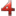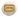Skeleton is a stock that appears in Modern Combat 4: Zero Hour.

## Modern Combat 4: Zero Hour

 Buffer Tube Carbon Compact Skeleton EAS Composite Tactical AMS

{{#infoboxbuilder: | 0:Theme = MC4 default | 0:ToggleContentLongerThan = 1000

| 1:Type = Title

```| 1:Value = Skeleton
```

| 2:Type = MainImage

```| 2:Value = File:MC4-Skeleton.png
```

| 3:Type = Line

```| 3:Label = Type
| 3:Value = Stock
```

| 4:Type = Line

```| 4:Label = Manufacturer
| 4:Value =
```

| 5:Type = Line

```| 5:Label = Based on
| 5:Value =
```

| 6:Value =

Statistics

| 7:Type = Line

```| 7:Label = Effect
| 7:Value =
```

| 8:Type = Line

```| 8:Label = Increases
| 8:Value = ?
```

| 9:Type = Line

```| 9:Label = Zoom
| 9:Value =
```

| 10:Type = Line

```| 10:Label = Decreases
| 10:Value = ?
```

| 11:Value =

Availability

| 12:Type = Line

```| 12:Label = Class
| 12:Value =
```

| 13:Type = Line

```| 13:Label = Tier
| 13:Value =
```

| 14:Type = Line

```| 14:Label = Unlocked
| 14:Value =
```

| 15:Type = Line

```| 15:Label = Cost
| 15:Value =4,500
```

| 16:Type = Image

```| 16:Value =
```

}} TBA